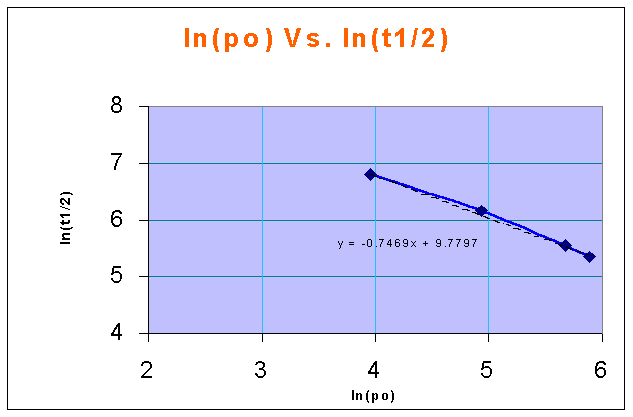### Half Life - Test Method

The thermal decomposition of nitrous oxide (N2O) in the gas phase at 1030 K is studied in a constant volume vessel at various initial pressures of N2O. The half-life data so obtained are as follows:


po (mm Hg)	52.5	139	290	360

t1/2 (sec)	860	470	255	212



2N2O -> 2N2 + O2

Determine the rate equation that fits the data.

Calculations:

t1/2 Vs. CAo in a log-log plot is a straight line with a slope of 1-n

CAo = pAo/RT = po/RT (for an ideal gas)

ln(CAo) Vs. ln(t1/2) in a linear XY plot gives a straight line, and the slope of the line is 1 - nfrom the above figure,

1-n = -0.7469

therefore, n = 1 + 0.7469 = 1.7469 = 1.75

i.e., Order of the reaction (n) = 1.75

t1/2 and CAo are related by the formula:

$$\displaystyle t_{1/2} = \frac{2^{n-1}-1}{k(n-1)}C_{Ao}^{1-n}$$

for a value of po = 290, and t1/2 = 255,

CAo = (290/760) x 1.01325 x 10-5/(8314 x 1030) = 4.515 x 10-3 gmol/lit.

therefore,

k = (21.75 - 1 - 1) x (4.515 x 10-3)(1-1.75) / (255 x (1.75 - 1)) = 0.2046 sec-1.(gmol/lit) -0.75

the rate expression for the given reaction is,

-rN2O = 0.2046 (C N2O)1.75